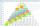# Angle in RT

Determine the size of the smallest internal angle of a right triangle whose sides constitutes sizes consecutive members of arithmetic progressions.

Result

x =  -90 °

#### Solution:Checkout calculation with our calculator of quadratic equations.

Leave us a comment of example and its solution (i.e. if it is still somewhat unclear...):

Showing 0 comments:Be the first to comment!#### To solve this example are needed these knowledge from mathematics:

Do you have a linear equation or system of equations and looking for its solution? Or do you have quadratic equation? See also our right triangle calculator. See also our trigonometric triangle calculator.

## Next similar examples:

1. AngleDetermine the size of the smallest internal angle of a right triangle which angles forming the successive members of the arithmetic sequence.
2. Trapezium internal anglesA trapezium where AB is parallel to CD, has angle A : angle D = 4 :5, angle B = 3x-15 and angle C = 4x+20. Find angle A, B, C and D.
3. Right triangleLadder 16 feet reaches up 14 feet on a house wall. The 90-degree angle at the base of the house and wall. What are the other two angles or the length of the leg of the yard?
4. Isosceles triangleWhat are the angles of an isosceles triangle ABC if its base is long a=5 m and has an arm b=4 m.
5. In a 2In a thirteen sided polygon, the sum of five angles is 1274°, four of the eight angles remaining are equal and the other four are 18° less than each of the equal angles. Find the angles. .
6. Ball gameRichard, Denis and Denise together scored 932 goals. Denis scored 4 goals over Denise but Denis scored 24 goals less than Richard. Determine the number of goals for each player.
7. Third memberDetermine the third member of the AP if a4=93, d=7.5.
8. AS sequenceIn an arithmetic sequence is given the difference d = -3 and a71 = 455. a) Determine the value of a62 b) Determine the sum of 71 members.
9. AP - basicsDetermine first member and differentiate of the the following sequence: a3-a5=24 a4-2a5=61
10. CalculateCalculate the length of a side of the equilateral triangle with an area of 50cm2.
11. Theorem proveWe want to prove the sentense: If the natural number n is divisible by six, then n is divisible by three. From what assumption we started?
12. Difference AP 4Calculate the difference of the AP if a1 = 0.5, a2 + a3 = -1.1
13. Linsys2Solve two equations with two unknowns: 400x+120y=147.2 350x+200y=144
14. Sequence 3Write the first 5 members of an arithmetic sequence: a4=-35, a11=-105.
15. SeatsSeats in the sport hall are organized so that each subsequent row has five more seats. First has 10 seats. How many seats are: a) in the eighth row b) in the eighteenth row
16. SequenceBetween numbers 1 and 53 insert n members of the arithmetic sequence that its sum is 702.
17. Arithmetic progressionIn some AP applies: 5a2 + 7a5 = 90 s3 = 12 Find the first member a =? and difference d = ?